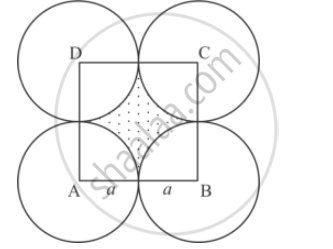Share

# Four Equal Circles Each of Radius A, Touch Each Other. Show that Area Between Them is 6/7a^2 - CBSE Class 10 - Mathematics

ConceptProblems Based on Areas and Perimeter Or Circumference of Circle, Sector and Segment of a Circle

#### Question

Four equal circles each of radius a, touch each other. Show that area between them is 6/7a^2

#### SolutionLet circles be with centres A, B, C, D]

Join A, B, C and D then ABCD is square formed with side = (a + a) = 2a

Area between circles = area of square – 4(area of quadrant)

= (2𝑎)2 − 4 (1/4𝑎𝑟𝑒𝑎 𝑜𝑓 𝑐𝑖𝑟𝑐𝑙𝑒 𝑤𝑖𝑡ℎ 𝑟𝑎𝑑𝑖𝑢𝑠 ′𝑎′)

= 4a^2 − 4 (1/4) × a^2

= 𝑎2(4 − 𝜋)

= 𝑎2 (4 −22/7)
= ((28−22)/7) a^2 =6/7a^2
∴ Area between circles =6/7a^2.

Is there an error in this question or solution?

#### Video TutorialsVIEW ALL 

Solution Four Equal Circles Each of Radius A, Touch Each Other. Show that Area Between Them is 6/7a^2 Concept: Problems Based on Areas and Perimeter Or Circumference of Circle, Sector and Segment of a Circle.
S